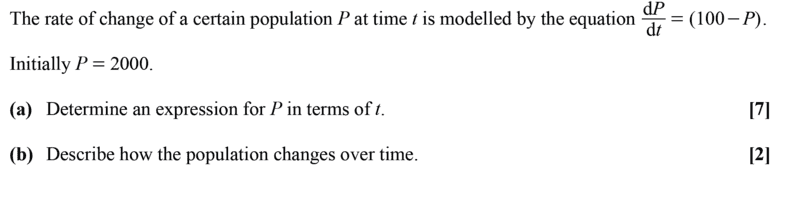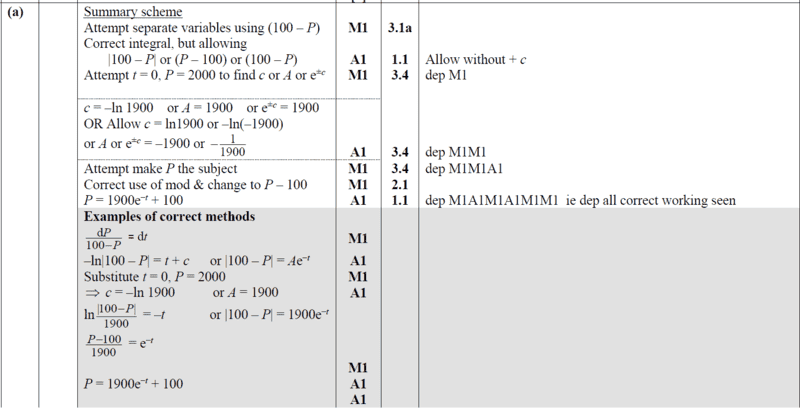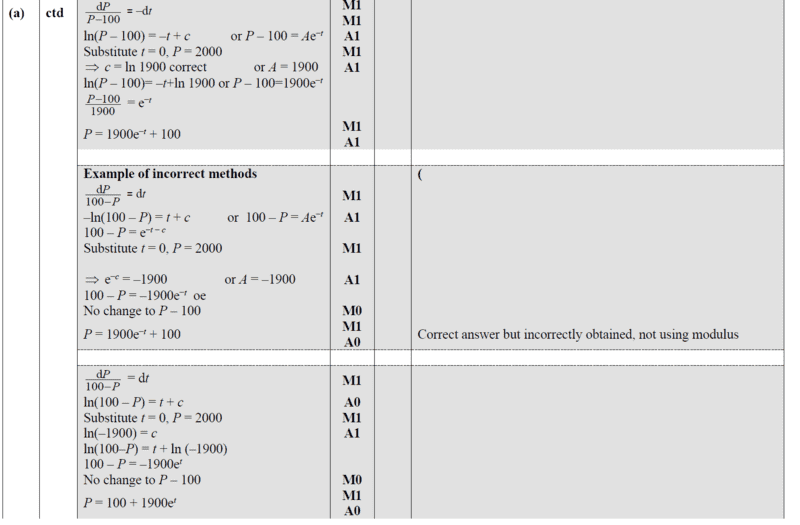# Solve the problem involving differential equation

• chwala

#### chwala

Gold Member
Homework Statement
See attached problem and ms guide
Relevant Equations
linear first order odeMy approach for part (a),

##\dfrac{dp}{dt}+P(t)=100##

I.f=## e^{\int 1 dt} = e^t##

Therefore,

##(e^t p)^{'}=100e^t##

##e^tp=\int 100e^t dt##

##e^tp=100e^t+k## Applying initial condition, ##p(0)=2000##

##2000=100+k##

##k=1900##

Therefore,

##e^tp=100e^t+1900##

##p=100+1900e^{-t}##

More insight is welcome guys...

•Delta2
I would just separate it: $$\begin{split} t &= \int_{2000}^{P(t)} \frac{1}{100 - p}\,dp \\ &= \left[ - \log|100 - p|\right]_{2000}^{P(t)} \\ &= -\log \frac{P(t) - 100}{1900}. \end{split}$$ (We expect $P(t) > 100$ because $P(0) > 100$.)

•Delta2
I would just separate it: $$\begin{split} t &= \int_{2000}^{P(t)} \frac{1}{100 - p}\,dp \\ &= \left[ - \log|100 - p|\right]_{2000}^{P(t)} \\ &= -\log \frac{P(t) - 100}{1900}. \end{split}$$ (We expect $P(t) > 100$ because $P(0) > 100$.)
Can you give me a more detailed proof of why ##P(t)>100##? @chwala solution solves it without the need to prove this...

Homework Statement:: See attached problem and ms guide
Relevant Equations:: linear first order ode

View attachment 302158

View attachment 302159

View attachment 302160

My approach for part (a),

##\dfrac{dp}{dt}+P(t)=100##

I.f=## e^{\int 1 dt} = e^t##

Therefore,

##(e^t p)^{'}=100e^t##

##e^tp=\int 100e^t dt##

##e^tp=100e^t+k## Applying initial condition, ##p(0)=2000##

##2000=100+k##

##k=1900##

Therefore,

##e^tp=100e^t+1900##

##p=100+1900e^{-t}##

More insight is welcome guys...
Do you know how to test your solution?
See if it satisfies the original equation.

Can you give me a more detailed proof of why ##P(t)>100##? @chwala solution solves it without the need to prove this...

$P(t) - 100$ is continuous; if it's strictly positive when $t > 0$, then there exists $T > 0$ such that $P(t) > 0$ for $0 \leq t < T$. It turns out that in this case there is no upper bound for $T$.

•Delta2
Another way to solve this is to use change of variables, ##Q(t)=P(t)-100##, hence the ODE becomes $$\frac{dQ}{dt}=-Q$$ which has the general solution ##Q(t)=ce^{-t}## e.t.c

•chwala
$P(t) - 100$ is continuous; if it's strictly positive when $t > 0$, then there exists $T > 0$ such that $P(t) > 0$ for $0 \leq t < T$. It turns out that in this case there is no upper bound for $T$.
You are not arguing accurately here, let me rephrase your argument a bit to what I believe is more accurate reasoning:
##P(t)-100## is continuous (because its derivative exist) and because ##P(0)-100=1900>0## therefore it exists an interval ##[0,T]## such that ##P(t)-100>0## for ##t\in [0,T]##. But it is not clear to me why there is no upper bound for T.

EDIT:OK I guess I get it now, let's hear what the OP has to say about this..

Last edited:
Do you know how to test your solution?
See if it satisfies the original equation.
Yes,
##⇒e^tp=100e^t+1900##

##e^tp-100e^t-1900=0.## Using implicit differentiation,

##e^tp+e^t\dfrac{dp}{dt}-100 e^t=0##

##e^t\dfrac{dp}{dt}=100e^t-e^tp##

##e^t\dfrac{dp}{dt}=e^t(100-p)##

##\dfrac{dp}{dt}=(100-p)##

•WWGD